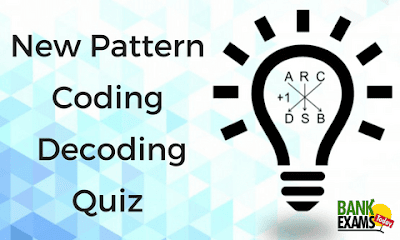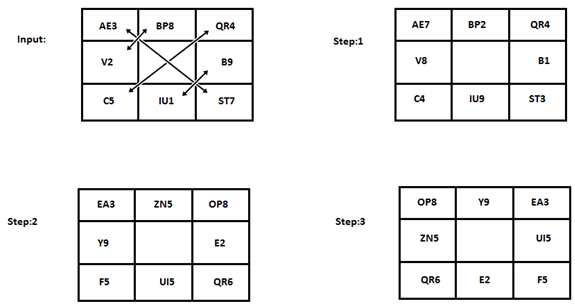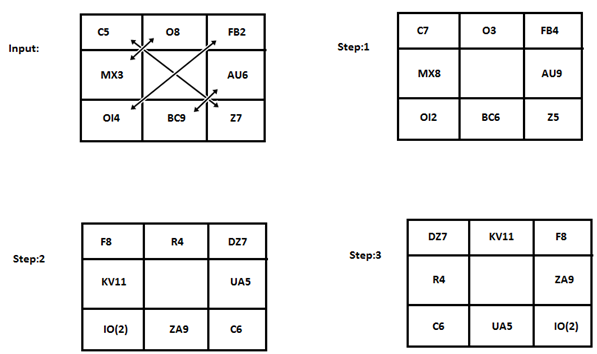# New Pattern Coding Decoding QuestionsDirection: Consider the following steps for given input and read the instructions to reach the last step:### Instructions:

Input to Step 1: Only alphabets are exchanged on the basis of arrows given.
Step1 to 2:
• If there are two consonant then change each alphabet with its second preceding alphabet and add 3.
• If there are two vowels then change their place with each other and less 4.
• If there is only one alphabet with a number then change the alphabet with its third succeeding alphabet and add 1.
Step 2 to 3: It also follows some pattern but is not same as of Step 1 & 2.### Question 1.

How many consonants are there in step 2?
A. 9
B. 7
C. 6
D. 8
E. 5
Ans. D.

### Question 2.

What is the total of all odd numbers in step 1?
A. 22
B. 23
C. 24
D. 21
E. 25
Ans. C.

### Question 3.

What will be the sum of all numbers in final step of given input?
A. 47
B. 52
C. 51
D. 42
E. 48
Ans. E.

### Question 4.

Which of the following represents the 2nd element in the first row of step: 2?
A. KV11
B. R4
C. DZ7
D. C.N.D.
E. ZA9
Ans. B.

### Question 5.

Which of the following represents the third element in 3rd row in step 1?
A. IO(2)
B. F8
C. C6
D. O3
E. None of these
Ans. E.

## Explanation:#### What's trending in BankExamsToday

Smart Prep Kit for Banking Exams by Ramandeep Singh - Download here